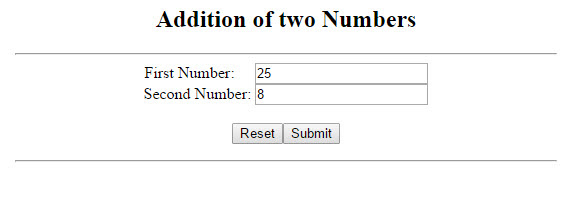# Replacing form fields by text in PHP

In PHP, the form fields can be replaced with custom text after processing by either using a html file, or hiding the form fields using the PHP script. The form fields will be replaced with custom text. This method can also used for applying static header and footer in the web page. In the following example, I have used HTML file and PHP script to add two numbers. The html file will be hidden during the execution.

Before ExecutionAfter ExecutionSource Code

form.html
<form method="post" action="<?php print $_SERVER['PHP_SELF']; ?>" > First Number: &nbsp;&nbsp;&nbsp;&nbsp;<input type="text" name="first"><br> Second Number: <input type="text" name="second"><br><br> <input type="reset" value="Reset"><input type="submit" value="Submit"> </form>  addition.php <html> <head> <title>Adding two numbers</title> </head> <body> <center> <br><br> <h2>Addition of two Numbers</h2> <hr width=40%> <?php if(!$_POST) {
include("form.html");
} else {
$n1 = ($_POST["first"]);
$n2 = ($_POST["second"]);
$n3 =$n1 + $n2; print "<br>Addition of$n1 and $n2 is$n3 <br><br>";
}
?>
<hr width = 40%>
</center>
</body>
</html>

Note: You must save both files in the same directory, and run the PHP file through the web server.